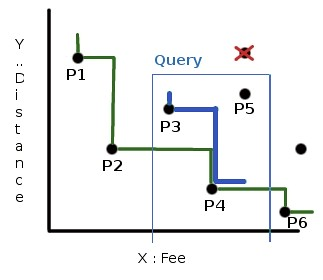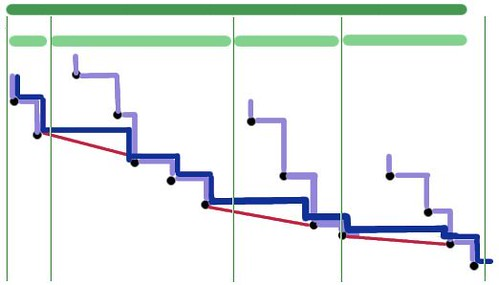# CKSCHOOL - Editorial

HARD

### EXPLANATION

This problem can be solved using a suitable data structure and it can be built to support online queries. There are at least a couple of ways to solve this, here we explain a solution using segment trees, which is simple to understand and answers each query in O(log2N). The most important step in solving this problem is, understanding the problem itself and abstracting it in a simple way. Imagine a 2D plane, where you mark a point for each school, taking fee on X axis and distance on Y axis, as shown in the figure below. Each query can be seen as a 3 sided range query, as shown by the thin blue line in the figure. The first step is to realize that, if we have many schools with the same fee, we can just keep the one among them having minimum distance and can completely ignore the other schools ( eg: the point striked off in red in the figure ). School X is preferred over school Y, if Y is strictly in the top-right quadrant, as seen from X. For example, P2 is preferred over P3 and P5. Now, how does the subset ‘Max Special S’ actually look like ? If you consider all the given points, then it contains the points on the green line {P1, P2, P4, P6}. The union of the top-right quadrants of these points cover all the points present. We refer this line as Special line.Note that the points that are not present in the global Special line can be part of a query special line, eg: P3 is not on the global Special line ( green color ), but is on the thick blue line, which is the Special line for the query shown in blue border. We sort all the points on X in increasing order and make them the leaves of our segment tree. Each internal node is associated with a set of points, which is the set of points present in the leaves of the subtree rooted at this internal node. We build the segment tree and store the Special line at each node, of the points associated with that node. To store the special line, we can just store the Y coordinates in the descending order and if the points are sorted already, we can just find the special line by doing a O(N) traversal at each node. Now, given a query (maxD, minF, maxF), we traverse the segmentree starting from root and with (minF,maxF) and identify the set of nodes that exactly covers the interval [minF, maxF]. We know that there can be at most O(logN) such nodes. Also, we have the Special lines computed already for each of them. All that is left is, to stitch these individual special lines, to get the special line for the given query.Consider two adjacent intervals [a,b] and [b+1,c]. If we have computed the Special lines for [a,b] and [b+1,c] already, can we find the Special line for [a,c] using them ? Yes, if you observe carefully, as we move from left to right, the points on the right side doesn’t influence the Special line for the points on the left side. So, the Special line of [a,c] starts exactly with the Special line of [a,b]. Lets say the special line of [a,b] = {Y11,Y12,…,Y1m} and that of [b+1,c] = {Y21,Y22,…,Y2n}. Take the y-coordinate of the last point on the special line [a,b] i.e., Y1m and find the largest y-coordinate on the special line of [b+1,c] that is smaller than Y1m and say its Y2k, then the special line for [a,c] = {Y11, Y12, …, Y1m, Y2k, Y2k+1, …, Y2n}. We can find this by a simple binary search for Y1m in {Y21,Y22,…,Y2n}. A picture is worth a million words, take a look at the figure on the right. The thick green interval is split in to some continuous light-green intervals, for which we have computed the individual Special lines already ( light-blue ). We start with the left-most interval and count its Special points and binary search for its last point ( shown with red line ) in the interval adjacent to it and count its special points starting from there. We continue this O(logN) search for each pair adjacent of O(logN) intervals, leading to O(log2N) per query.

### SETTER’S SOLUTION

Can be found here.

//